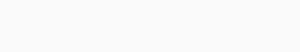# If you can’t solve these 2023 BECE Mock Questions, You are not ready for the BECE## If you can’t solve these 2023 BECE Mock Questions, You are not ready for the BECE.

### [60 MARKS]

Answer only four questions in all.

1. a) In a class of 47 pupils, 25 study Mathematics and 32 study English.

8 pupils do not study any of the two subject.

1. Illustrate this information on a venn diagram.
2. How many pupils study only one subject.
3.     Simply the expression      –
4.     Find the value of y in the diagram below.b. Factorize completely the expression   2ap – aq – bq + 2bq.

c) A water tank in a form of a cuboid of height 22cm and a rectangular base of length 7cm and width 5cm is filled with water. The water is then poured into a cylindrical container of diameter 14cm. Calculate the height of the water in the cylindrical container. [Take  = ]

d) Francis bought 160 copies of books at GH¢ 3.50 per copy. He sold each of them at GH¢ 4.30. Find

i. the total cost price

ii. his percentage profit

If you can’t solve these 2023 BECE Mock Questions, You are not ready for the BECE

3. The circumference of a circle is 44cm. Find it’s area.1. The marks obtained by 40 pupils in a test were as follows:

4   8   7   6   2   1   8   7   1   7

1   7   4   3   7   3   1   2   3   8

6   4   7   5   2   2   4   6   2   5

7   5   4   8   3   7   3   5   4   1

If you can’t solve these 2023 BECE Mock Questions, You are not ready for the BECE

1. Construct a frequency distribution table for this data
2. What is the mode of distribution?
• Calculate the mean mark.
1. What percentage of the pupils passed, if the pass mark is 5.
2. What is the probability that a pupil selected at random scored not more than 4 marks.

1. a) Given that p =  and q = , find the values of x and y if p = ½ qIf you can’t solve these 2023 BECE Mock Questions, You are not ready for the BECE1. Consider the diagram below representing a field PQURST with a circular pond of diameter 14m. find
• the distance round the field
• the area of the field excluding the pond